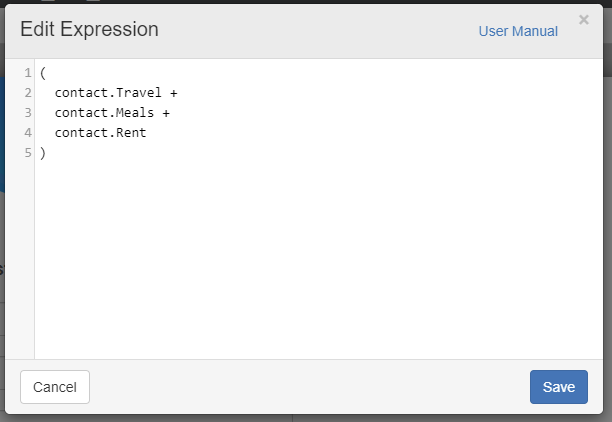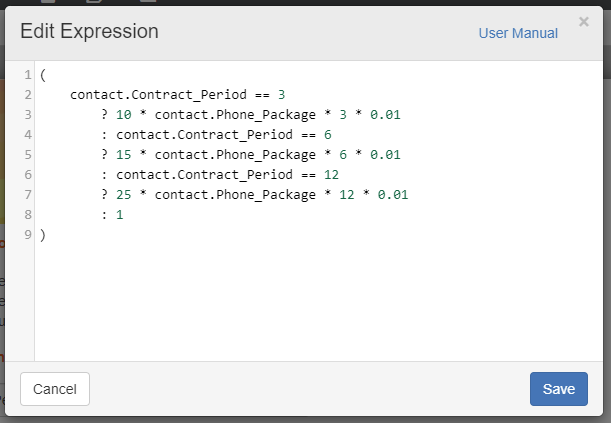Follow

# Calculation & Expression Examples

In the previous article, we provided background for using Calculations. Here we will show some examples to illustrate their usage and value. (Note: being familiar with JSON coding is an advantage here).

Here is an example of a basic Calculation, which shows 3 Fields from the Contact List (Travel, Meals and Rent), and shows how to sum up those 3 Fields. The result is a 'Total Expenses' value, calculated by the expression here and whatever values are entered for each field in the webform.Here is a more complicated Expression, which deals with a discount based on different Phone Package and Contract Period options chosen. The result is a percentage amount shown which is discounted from the overall total.Looking at the first line and understanding the star * symbol as multiplication, we can see that if 'Contract_Period' equals 3: the number 10 is multiplied by the 'Phone_Package' option chosen, and then by 3 and finally by 0.01 (to get the %).

The same thing happens if 6 or 12 is chosen for the 'Contract_Period' option.